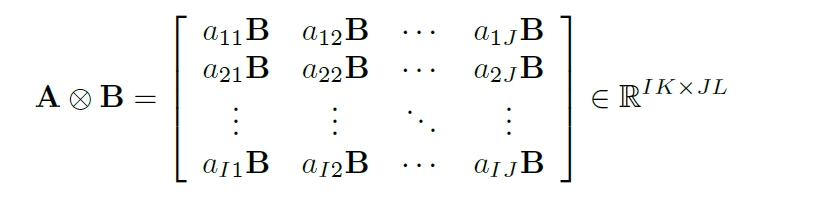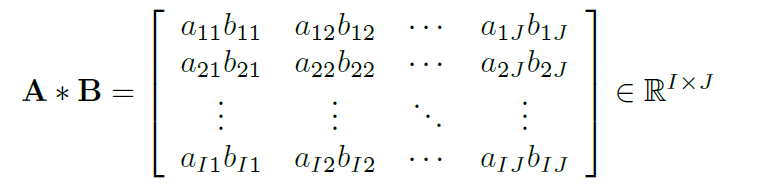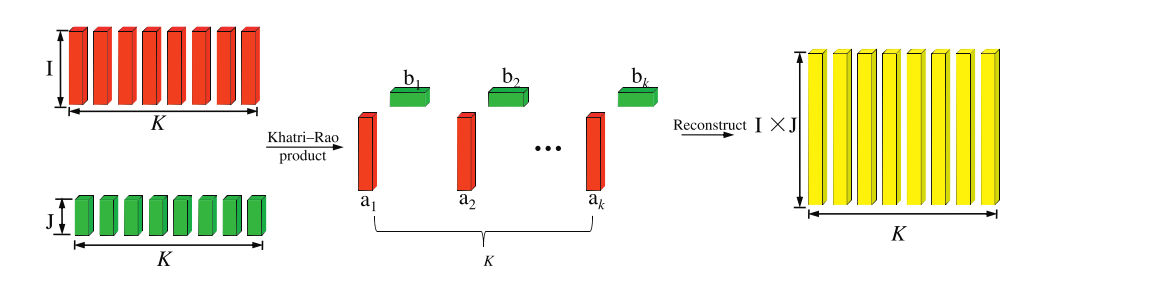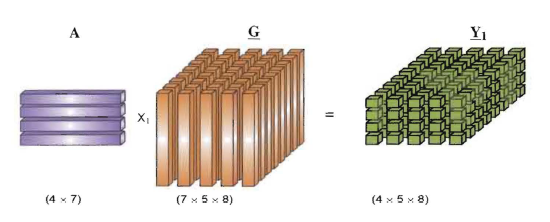## 张量定义及运算

### Kronecker乘积(Kronecker Product)

Kronecker乘积定义在两个矩阵${\mathbf{A}} \in {\mathbb{R}^{I{\mathbf{ \times }}J}},{\mathbf{B}} \in {\mathbb{R}^{K{\mathbf{ \times }}L}}$上的运算:Hadamard 乘积定义在两个相同大小的矩阵${\mathbf{A}} \in {\mathbb{R}^{I{\mathbf{ \times }}J}},{\mathbf{B}} \in {\mathbb{R}^{I{\mathbf{ \times }}J}}$上的运算:### Khatri-Rao乘积(Khatri-Rao Product)

Khatri-Rao乘积定义了两个相同列数的矩阵${\mathbf{A}} \in {\mathbb{R}^{I{\mathbf{ \times }}K}},{\mathbf{B}} \in {\mathbb{R}^{J{\mathbf{ \times }}K}}$上的操作,它的过程如下图所示。 ${\mathbf{A}} \odot {\mathbf{B}} = \left[ {\begin{array}{*{20}{l}}{{{\mathbf{a}}_1} \otimes {{\mathbf{b}}_1}}&{{{\mathbf{a}}_2} \otimes {{\mathbf{b}}_2}}& \cdots &{{{\mathbf{a}}_K} \otimes {{\mathbf{b}}_K}}\end{array}} \right]\in {\mathbb{R}^{IJ{\mathbf{ \times }}K}}$### 张量与矩阵的模积(Mode-n Product)### 张量与向量的模积(Mode-n Product)

${\left( {X{{\bar \times }_n}{\mathbf{v}}} \right)_{{i_1} \cdots {i_{n - 1}}{i_{n + 1}} \cdots {i_N}}} = \sum\limits_{{i_n} = 1}^{{I_n}} {{x_{{i_1}{i_2} \cdots {i_N}}}{v_{{i_n}}}}$